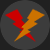Share via:

PHP: While and For Loops

Tutorial on how to use the while and for loops of PHP.

1102 viewsEdited: 2019-09-11 16:08While and For loops can be useful for a variety of tasks, one of which is to generate content automatically. For instance, the navigation links on a website can be generated using a simple loop.

Typically, loops will continue running until a given condition has been met. It can, however, be difficult to create very long loops, depending on your server configuration. Many servers have a maximum execution time, after which the script will be stopped, regardless if it has finished or not.

A simple while loop is shown below:

\$number = '1';

while (\$number = '1') {
echo 'Repeating this Forever';
}

While Loop

The below will continue for as long as \$number is equal to 1.

The while loop syntax is: while (condition) {DoStuff}

It will check to see if the condition is met, at the beginning of each run.

\$number = '1';

while (\$number = '1') {
echo 'Repeating this Forever';
}

Lets limit the loop to a maximum of 15 runs.

\$number = '1';

while (\$number <= '15') {
echo 'Run Number:' . \$number . "\n";

++\$number;
}

The conditions basically says, for as long as \$number being less then, or equal to 15, run the loop. The last part ++\$number;, will add 1 to \$number at the end of each run.

Be sure not to make below mistake:

while (\$number <= '15') {
\$number = '1';
echo 'Repeating this Forever' . "\n";

++\$number;
}

Since it will reset \$number each time the loop has executed. Also make sure not to declare variables inside the loop unless its necessary, since doing so will re-declare them each time the loop is executed, and as such slow down your scripts.

For Loop Example:

For loops are difficult for many beginners to learn, one reason might be the "for" which apears confusing. So i ask that you keep in mind the While Loop Example from before, then you will have an easier time understanding the next example.

for (\$number = '1'; \$number <= '15'; ++\$number) {
echo 'Run Number:' . \$number . "\n";
}

The for loop actually uses the same order. However instead of declaring \$number before the Loop, and adding 1 to \$number inside the "actions list", we simply include those actions in the syntax of the loop.

The for loop Syntax is: for (expr1; expr2; expr3) { DoStuff }

Expr1 is where i declared \$number, expr2 can be translated into the "condition" from the while loop, and finally expr3 is the action to be performed after each run, in this case, the addition to \$number.